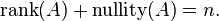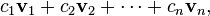# Blog Archives

## 2012 in review

The WordPress.com stats helper monkeys prepared a 2012 annual report for this blog.Here’s an excerpt:

About 55,000 tourists visit Liechtenstein every year. This blog was viewed about 250.000 times in 2012. If it were Liechtenstein, it would take about 5 years for that many people to see it. Your blog had more visits than a small country in Europe!

## Dimension

The dimension of the column space is called the rank of the matrix. The rank is equal to the number of pivots in the reduced row echelon form, and is the maximum number of linearly independent columns that can be chosen from the matrix. For example, the 4 × 4 matrix in the example above has rank three.

Because the column space is the image of the corresponding matrix transformation, the rank of a matrix is the same as the dimension of the image. For example, the transformation R4 → R4 described by the matrix above maps all of R4 to some three-dimensional subspace.

The nullity of a matrix is the dimension of the null space, and is equal to the number of columns in the reduced row echelon form that do not have pivots. The rank and nullity of a matrix A with n columns are related by the equation:This is known as the rank-nullity theorem.

## Column space

Let A be an m × n matrix, with column vectors v1v2, …, vn. A linear combination of these vectors is any vector of the formwhere c1c2, …, cn are scalars. The set of all possible linear combinations of v1,…,vn is called the column space of A. That is, the column space of A is the span of the vectors v1,…,vn.

Example
If, then the column vectors are v1 = (1, 0, 2)T and v2 = (0, 1, 0)T.
A linear combination of v1 and v2is any vector of the formThe set of all such vectors is the column space of A. In this case, the column space is precisely the set of vectors (xyz) ∈ R3 satisfying the equation z = 2x (using Cartesian coordinates, this set is a plane through the origin in three-dimensional space). Read the rest of this entry

## PYTHAGORAS GOES TO POWER POINTHEM,, tak asing lagi kita kenal yang namanya pythagoras.. hahaha

sebuah materi yang udah pernah kita pelajari pada waktu kelas 8 di SMP..

yah, karena seiring juga dengan tehnologi IT yang sudah berkembang pesat tentunya, kemasan materi pun jg harus bisa mengimbangi dong. makanya ini tak postingkan tentang power point sebuah pythagoras.. semoga bermanfaat… Amin

PYTHAGORAS